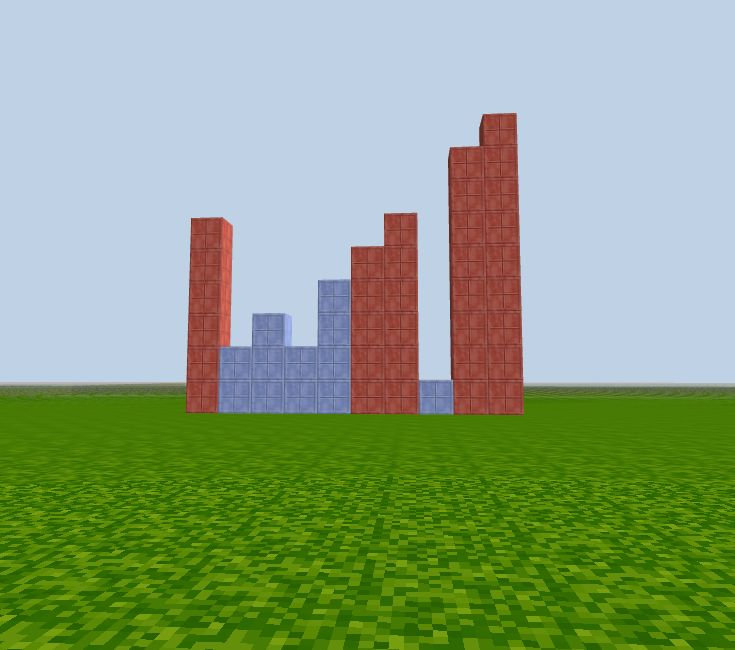# Ternary Operator '?'

Sometimes we need to assign variable value depending on a condition, for example:

``````let permission;
let age = Number(prompt('How old are you?'));

if (age > 13) {
} else {
permission = "Sorry, you are not old enough to enter";
}

console.log(permission);
``````

JavaScript has a conditional(ternary) operator to assign a variable depending on a condition.

### Ternary Operator Syntax

``````variableName = (condition) ? value1 : value2
``````

If the condition is `true`, take `value1`, else take `value2`.

We can do the above example in a simpler way:

``````let age = prompt('How old are you?');

let pass = (age > 13) ? "Yes, please enter." : "Sorry, you can not enter.";

console.log(pass);
``````

### CodeCraft Visual

Build columns representing a number array. Based on a condition, we can build different color columns for different numbers:

##### Bigger than 5?

If the number is smaller than 5, use `'box_blue'`, else use `'box_red'` as the material value.

``````let arr = [3,5,2,8,10, 4,6];
for (let i = 0; i < arr.length; i++) {
let material = (arr[i] < 5) ? 'box_blue' : 'box_red';
column(i, -20, arr[i], material);
}
``````##### Even or Odd?

If we change the condition: `let material = (arr[i] % 2 == 0) ? "box_blue" : "box_red";`

then we can build blue columns for even numbers and red columns for odd numbers.